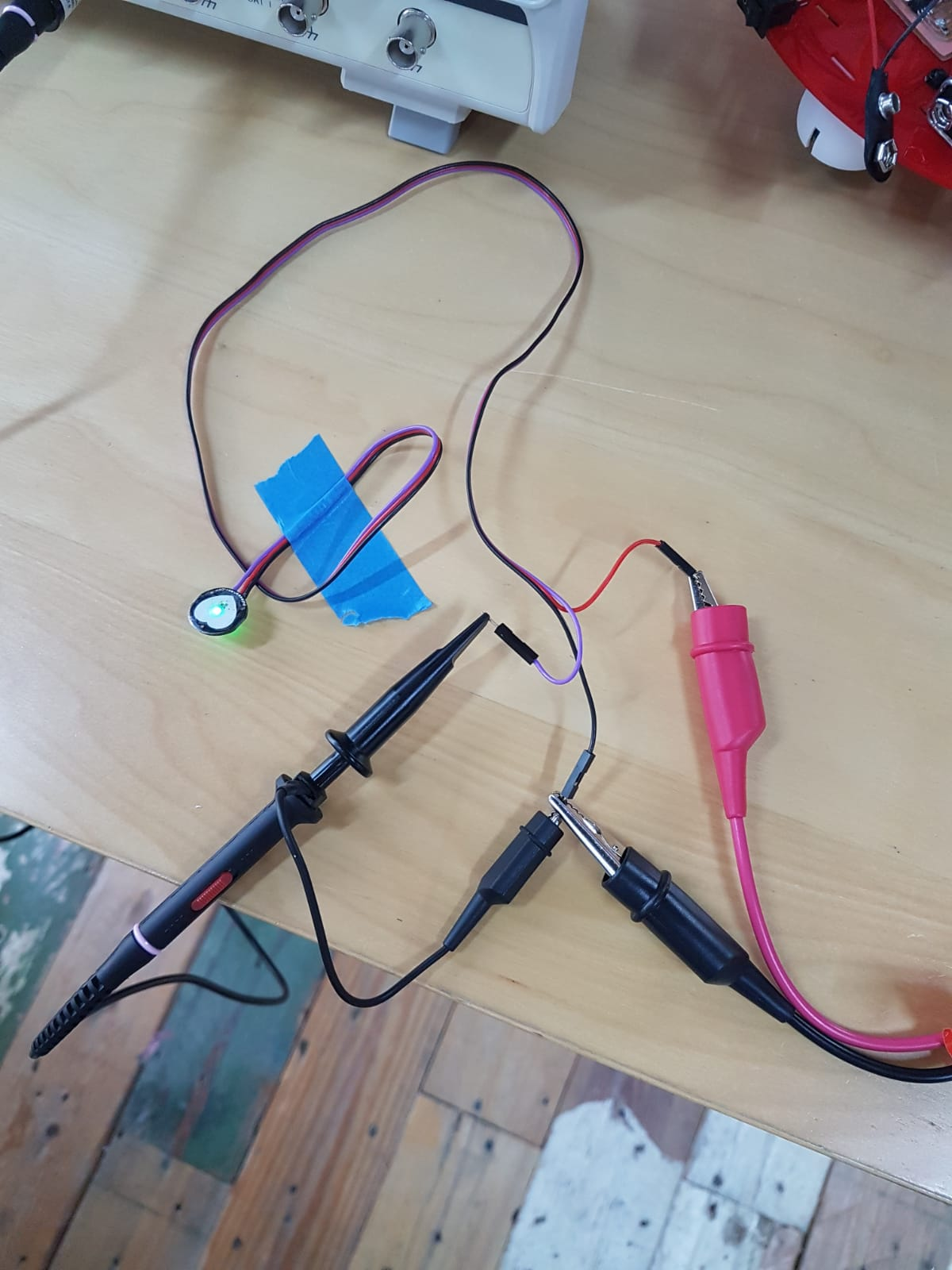# Input devices¶

This week’s group assignment is to Measure the analog levels and digital signals in an input device

## Temp sensor¶

We measured the analog value of a Temperature sensor using a multimeter. A multimeter is an electronic measuring instrument that combines several measurement functions in one unit. A typical multimeter can measure voltage, current and resistance. The analog input device we are measuring using the multimeter is a Low Voltage Temperature Sensor TMP36 .

As per the datasheet, we will find that it need to be powered by a voltage of (2.7 V to 5.5 V) and is calibrated directly in degrees celcius. Meaning the values we get with a simple conversion coorisponde to celcieus degree values.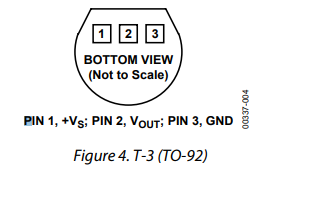The first step is to connect the tempreture sensor to the supply voltage. The data sheet shows the correct way to connect. The data sheet also has a graph showing the voltage level at every tempreture. form this graph we can conclude that the tempreture in celcieus equals 100*(reading in V) - 50.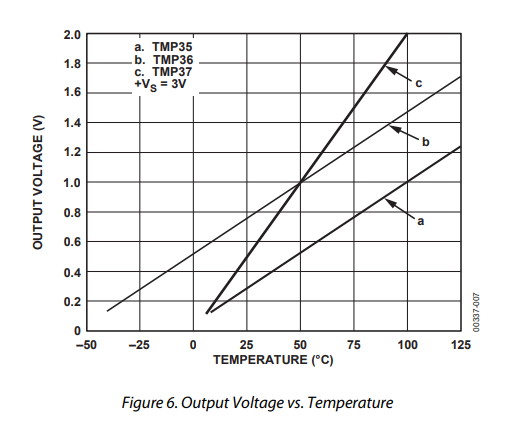I powered on the tempreture sensor using Arduino board and connected the data pin & ground to the multimeter. I set the multimeter to the VDC setting.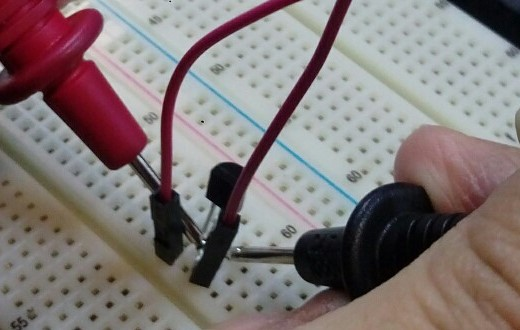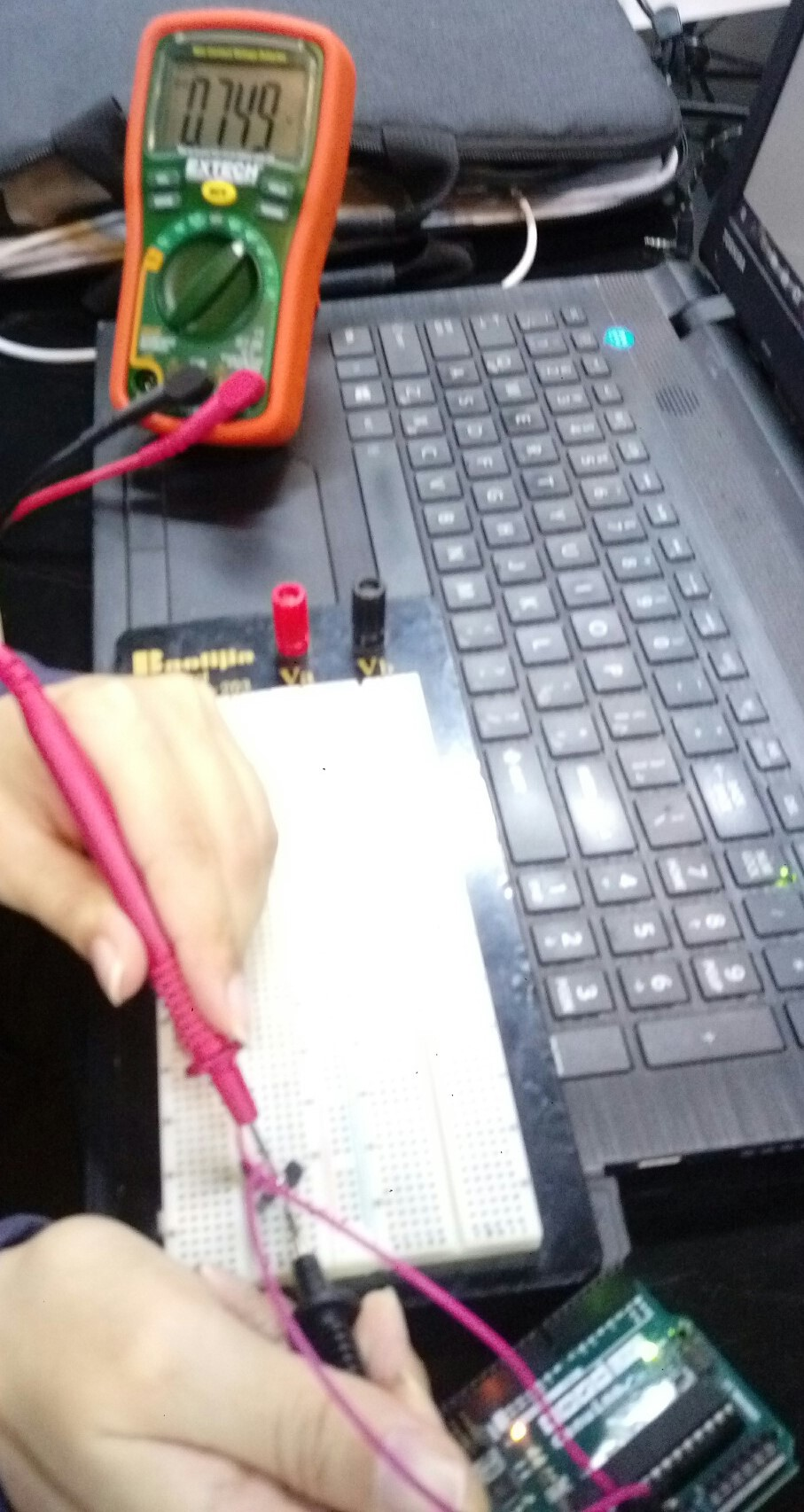The voltage is 0.749V, lets calculate the tempreture. Temp = (0.749 * 100 ) - 50 = 24.9 degrees celcius, which is around room tempreture so it makes sense. I touched the tempreture sensor with my hand & the voltage started increasing because of my hands heat.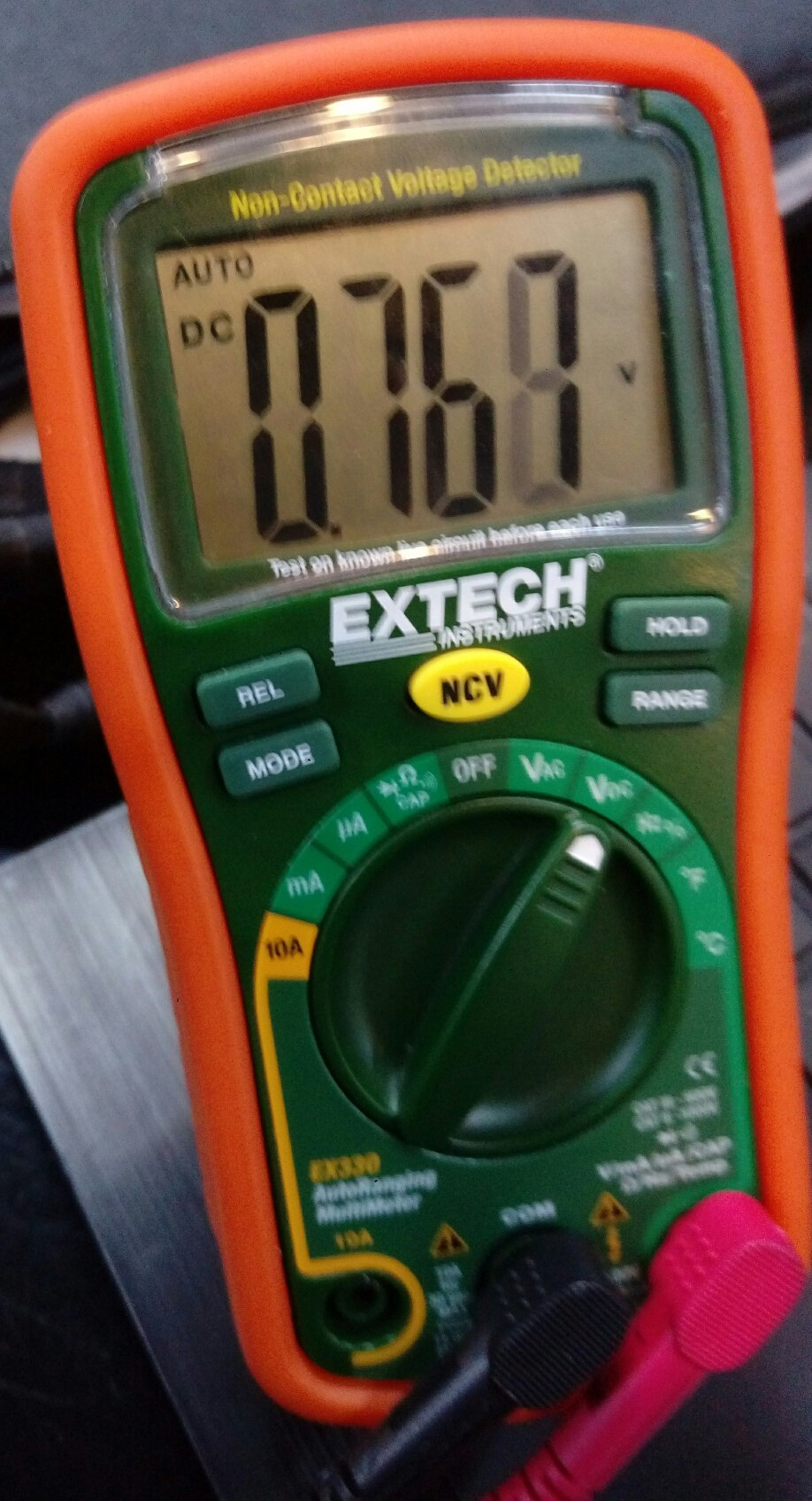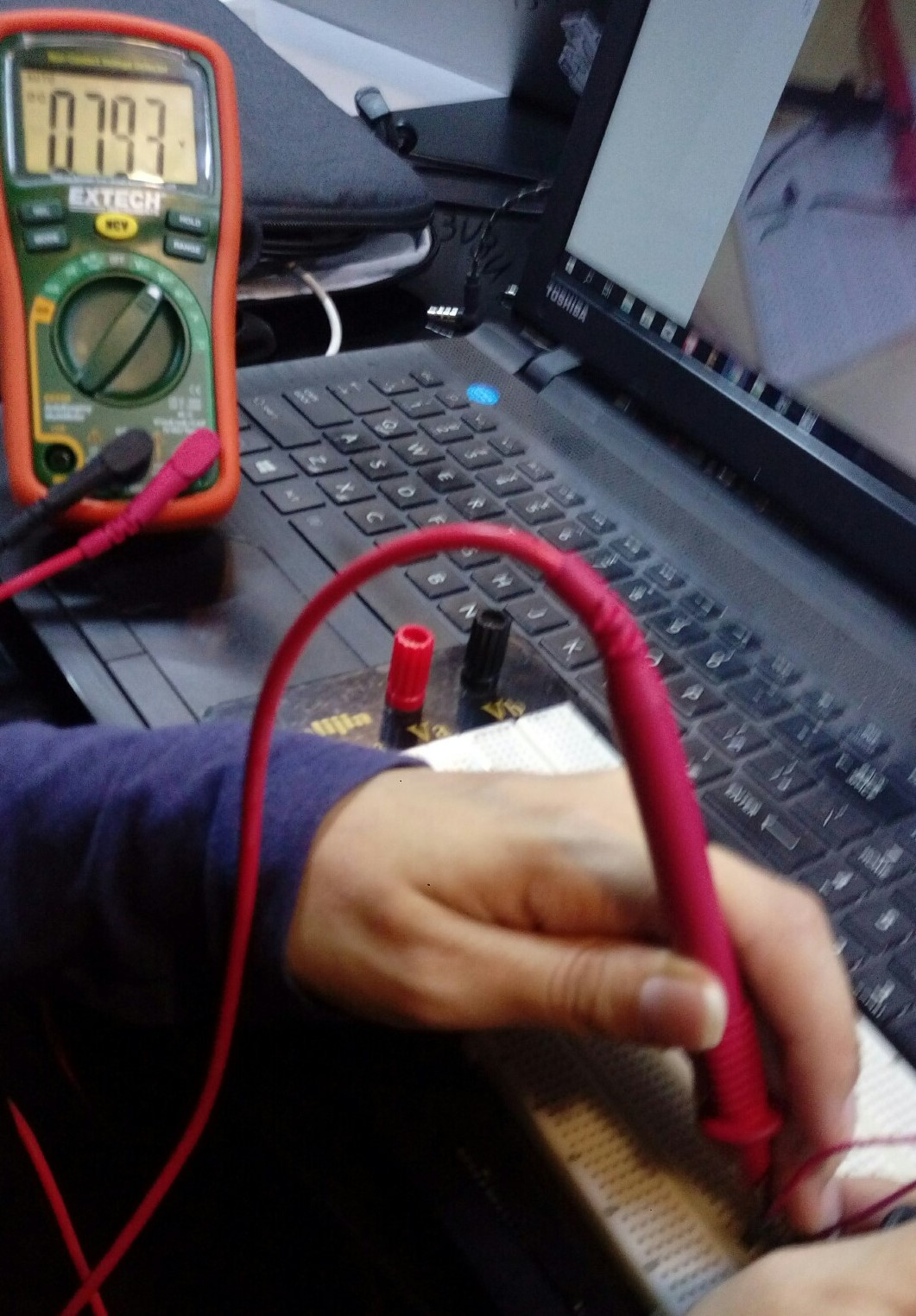It reached 0.793 v.

Temp = (0.793 * 100 ) - 50 = 29.3 degrees celcius

## Heart beat sensor¶

We connected a heart beat sensor to the power supply at 5v then we connected the osiliscope to the GND terminat and the othe wire to the heart beat sensor signal wire.